```|Portada|Blog|Space|
```
```                            sed-circuit-simulator

a digital circuit simulator written in sed.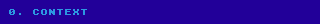As many of you might know, one of my hobbies is writing code on a programming
language called "sed", commonly available on unix-like operating systems.
Most unix users developers only have a superficial knowledge of this programming
language, let alone developers in general, and with good reason: doing anything
other than a simple "s/pattern/replacement/" substitution is a recipe for an
unreadable mess.  In other words "sed" is a write-only or esoteric programming
language.

Sed only contains two places were we can store a string: the pattern space and
the hold space.  The program in its most basic form will be executed once per
each input line, and each execution will start with the pattern space containing
such line, the hold space however is kept in memory across the all the input,
and in this case we will certainly be using that space.

This means we have to:
* Encode all the memory structures of our program into a single string using
a format that simplifies the regexp replacements we will be using.
- HINT: To do this, reserving a subset of the characters for usage of these
structures is in general a good idea.
* Validate all input so that we either:
* Reject lines with reserved characters.
* Remove reserved characters from the input.
* Escape those characters: ie: html uses «&quot;» for «"», and then «&amp;»
for «&».
* Consider having a partial order for the parts of the string, as that can
be useful for marking whether some processing has been done, for example
a given delimiter could separate the processed parts from the rest.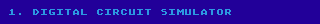To reduce the scope of this simulator we will be considering circuits as just
a bunch of logic gates connected by cables:
* a cable can be on or off (which we represent by 1 or 0 respectively),
* logic gates are boxes with one or many inputs and one output whose value
depends on whether the inputs are on or off.  Even though in real life
there are many types of logic gates, here we will only be supporting the
following: OR, AND and NOT.
* an OR gate outputs 1 if any of its inputs are 1, otherwise it outputs 0.
* an AND gate outputs 1 if all of its inputs are 1, otherwise it outputs 0.
* a NOT gate has only one input and the output is the input negated,
that is 1 if the input is 0; and 0 if the input is 1.
* we don't allow outputs connected together, as in some contexts that could
cause a short circuit on real life (it's quite more complex in reality, as
there are ways to support multiple outputs on a single cable, like using
three-state or open collectors).

So in order to model this our simulator model circuits as a bunch of nodes,
where a node has a definition and a state.
* The state of all nodes is initially set to 0, and at each step we
recalculate the state of each node based on the states of the nodes at
the previous step.  This means that each node will represent an output with
a propagation delay of one step.
* Definitions are mathematical expressions which can contain:
* Node names (all of them must be defined).
* The binary «+» operator returning the OR of the expressions.
* The binary «*» operator returning the AND of the expressions.
* The unary «!» operator returning the NOT of the expression at its right.
* Balanced parenthesis, to override the order for the expression evaluation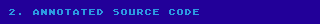Feel free to add any 'p' commands in the source code to show the
intermediate state at any place.

1│ s/ //g; /^\(<[^>|:=+*()!]*:[^>|:=]*>\)*\$/!{s/.*/syntax error/;n;}
2│ 1s/^/|/; x; G; s/\n//; s/.*|\(.*\)/\1&/
3│ :d; s/\(<[^>]*\):[^>]*\(>.*|\)/\1=0\2/; td
4│ :a; s/\(.*\)\(<[^>]*[=:]\)[^>]*>\([^|]*\)\2\([^>]*>\)/\1\2\4\3/; ta
5│ s/|.*/&&/
6│ :v; s/\(<\([^=]*\)=\(\)>.*|.*|.*[:+*!(]\)\2\([+*)>]\)/\1\3\4/; tv
7│ h; s/.*|//
8│ :e
9│   s/!0/1/g; s/!1/0/g; s/(\(\))/\1/g; te
10│   s/1\*1/1/g; te; s/\*/0/g; te
11│   s/0+0/0/g; te; s/+/1/g; te
12│ /:[^01]\|:.[^>]/{s/^/eval error: /;q;}
13│ s/:/=/g; x; s/[^|]*\$//; G; s/.*\(|.*\)|.\(.*\)/\2\1/; h; s/^/-- /

Decoding each line:

1. Remove spaces from the input, each input line should have the following
format: 0 or more assignments where each assignment is "<" + the name of
the variable being assigned + ":" + the definition for that variable + ">".
Otherwise we show "syntax error" jumping to the beginning of the program
to process the next line.  Among the names and definitions we reject any
occurrences of the characters ­«|:=<>», these will be reserved characters.

2. Let's try to make sense of this line by converting it to lua.  Note as
well that in the line 8 we are overwriting the hold space, hold space we
don't use until the very last line, so really we don't care about what's
left in there after this line.  So:
-- We add a pipe if we are reading the first line (that's the meaning
-- of the 1 before the 's' command):
if first_line then
pattern = '|' .. pattern
end
-- With 'x' we interchange the hold and pattern spaces:
pattern, hold = hold, pattern
-- and with the 'G' command we append a new line plus the hold space to
-- the pattern space.
pattern = pattern .. '\n' .. hold
-- We delete the newline character:
pattern = pattern:gsub('\n', '')
-- And then add whatever is after the pipe, to the beginning of the line:
pattern = pattern:match '.*|(.*)' .. pattern

Note that what we really intended by doing «x;G;s/\n//» is to just have:
pattern = hold .. pattern; but there's no command in sed for this.

In other words, since we don't care about the hold space, this would be the
equivalent to just the pattern space if we combine all these commands:
if first_line then
pattern = pattern .. '|' .. pattern
else
pattern = hold:match'.*|(.*)' .. pattern .. hold .. pattern
end

After this line we will have two sections separated by a pipe: on the left
we will be ultimately storing the current state of the circuit, on the
right all the definitions of each node.

3. This line is in charge of initializing the states of newly added variables
to 0.  We can identify the new variables because they have a colon in their
definition.  Note that here we only do replacements on the left part of the
pattern space, that's why we need to reach the pipe at each replacement.

4. Here we add support for redefining nodes, if the same name is defined twice
in the same section we will take the value of second definition and place
it in the first one while deleting the second definition.  We do it this way
to maintain the order of the definitions, since it's way easier to read the
output if the order of the variables doesn't change.

Exercise 1: look at the regexp and notice that it begins with \(.*\),
obviously corresponding to \1, yet the only place where we use \1 is at
the beginning of the replacement string.  Try to explain why we added
this seemingly useless group.  The answer is at the end of the blog post.

5. This short line requires a bit more explanation, here we are duplicating
the second section into a new third section.  We will see why we do this
in the next line once we start using it.

6. Now we replace each and every one of the referenced variables in the
third section by the values on the state's first section, after this is
complete we expect to have the third section as just a bunch of formulas
with zeros and ones like this:

<d:1><e:0><g1:!(1*1)><g2:!(1*0)><g3:!(0*!1)><q:!(0*1*1)>

7. So, we save everything in the hold space and leave only this third section
in the pattern space.

8. Because from this point we will be evaluating these expressions ensuring
the precedence order of the operators.  After each successful replacement
we will go back to this label.

9. With the highest priority we have the NOT operator !0 is 1 and !1 is 0,
and the parenthesis that contain just a value (1) and (0)... pretty
straightforward replacements.

10. Then it comes the ANDs: 1*1 first so that we can have a simple \*
for the rest of the AND cases.

11. Similar for OR: 0+0 -> 0 first, then + -> 1.

12. Calculated expressions should end up being :0> or :1>, otherwise it's
either a syntax error (or we forgot to define a variable).

13. Let's save the newly calculated state by changing colons to equal sign,
swapping the spaces, removing the old third section, appending a newline
and the new state.  Then it comes s/.*\(|.*\)|.\(.*\)/\2\1/ which results
in the new state, a pipe and the definitions.  Note that the period after
the second pipe is the one matching the newline.

We save that line and prepend a few dashes to display the output and get
ready to process the next line.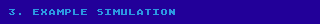In this example we will be doing an Earle latch:

https://en.wikipedia.org/wiki/Flip-flop_(electronics)#Earle_latch

We then define the circuit as two inputs, d: data, e: write enable, and four
NAND gates: g1, g2, g3 and q:

<d:0><e:0> <g1:!(d*e)><g2:!(d*q)><g3:!(q*!e)><q:!(g1*g2*g3)>

This flip flop has the nice characteristic that the input to output propagation
is constant given that the clock "e" (write enable) and its opposite "!e" are
synchronized.  Since the "e" negation is done directly in the gate g3, we don't

The multiple panels here, show the state of the circuit after each step of
propagation delay.  Even though the gates seems to be returning the incorrect
value we should remember that gates here take one step each to update their
value: they are computed based on the state of the circuit at the previous
step.

Note that with the inputs initialized at 0, this results in the final output
q oscillating between 0 and 1.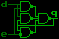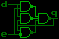We reset the latch by setting the write enable input to 1 for a couple of steps.

<e:1>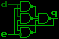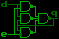<e:0>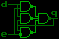Now we will change the latch value by setting d now to 1, together with the
write Enable input for a couple of steps.

<d:1><e:1>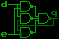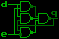<e:0>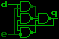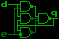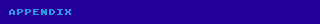In language theory we usually define grammars as just the set of strings
matched by them without considering *how* or *what part* of the string is
matched, as that doesn't affect whether it matches or not.

When replacing this DOES matter, so this is how regexps are matched in sed:
* The first lexical element of a regex tries to match the earliest possible
occurrence.  IE: s/x/<X>/ changes axbxc to a<x>bxc
* The first lexical element of a regex tries to find the longest possible
match.  IE: s/foox*/!/ changes aafooxxxxaa to aa!aa
Note that the first rule will have priority: this is why the replacement
s/o*/!/ changes foo to !foo (/o*/ matches at the beginning with 0 o's)
* In case of the alternative operator «\|» sed tries to match the earliest
longest matching, no matter if it is the first or second operand matching.
IE: s/f\|fo/!/ changes fox to !x
* In case of multiple lexical elements the first will receive priority on
these rules: s/\([ab]*\)\([bc]*\)/<\1><\2>/ replaces abc with <ab><c>

That's it.

Then why does the \(.*\) at the start matters? because by adding that, we place
a different first lexical element which tries to match as much text as
possible, making the rest of the replacement match the latest possible part
of the regex.

Take for instance the following replacement:
s/\(<[a-z]*>\)\(.*\)\(<[a-z]*>\)/L:\1\2R:\3/

If the input is <a> <b> <c>, the resulting output would be L:<a> <b> R:<c>.

Doing something like this be ok in many cases but not in the line 4 of the
code, because what we wanted to do there was to retain the last definition.
<a:d1>...<a:d2>...<a:d3> would first change to <a:d3>...<a:d2>... and then to
<a:d2>......, leaving the middle one.  We can either match the first two or
the last two, it would be ok either way, and as we have just seen it's very
easy to match the last two by adding a \(.*\) group at the beginning.

As an extra exercise try to rewrite the regex in line 4 of the code to match
the *first* two definitions instead.  Good luck!.  If you manage to do it,
please write a comment with the response.  Hint: I'm sorry to inform you that
GNU sed does not support non-greedy matches: «.*?»

.utterances {
margin-left: 0px;
filter: brightness(90%);
max-width: 640px;
transform-origin: top left;
transform: scale(.8);
}
```
```document.title="wiki: blog/sed-circuit-simulator"
```